1. When a body is accelerating, the resultant force acting on it is equal to its

2. A toy cannon is mounted vertically on a cart. The cart is moving along a straight-line with constant speed. A spring inside the cannon shoots a ball vertically upwards.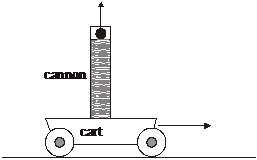No resistance forces act on the cart and on the ball. Which one of the following statements is true about the position where the ball will land?

3. Mandy stands on a weighing scale inside a lift (elevator) that accelerates vertically upwards as shown in the diagram below. The forces on Mandy are her weight W and the reaction force from the scale R.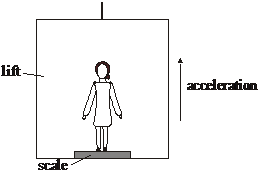The reading of the scale is

4. A body of weight 2W hangs vertically from a string attached to a body of weight W. Weight W is released and both bodies fall vertically.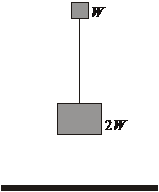Air resistance may be neglected. What is the tension in the string during the fall?

5. An object is moving at constant velocity. Which one of the following quantities must have zero magnitude?

6. Two blocks having different masses slide down a frictionless slope.Which of the following correctly compares the accelerating force acting on each block and also the accelerations of the blocks down the slope?

 Accelerating force Acceleration A. Equal Equal B. Equal Different C. Different Equal D. Different Different

7. . If the resultant external force acting on a particle is zero, the particle

8. A ball of weight W is dropped on to the pan of a top pan weighing balance and rebounds off the pan.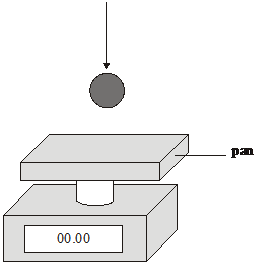At the instant that the ball has zero velocity when in contact with the pan, the scale will read

9. A particle moves under the influence of a force F. The graph below shows the variation of the force F with the distance d moved by the particle.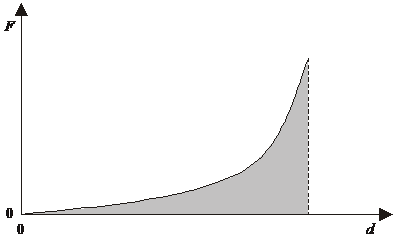The shaded area is equal to

9. A particle moves under the influence of a force F. The graph below shows the variation of the force F with the distance d moved by the particle.The shaded area is equal to

Question 1 of 10